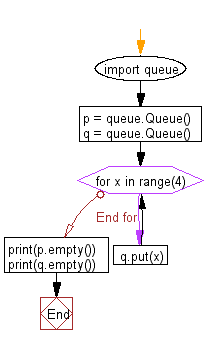﻿ Python heap queue algorithm: Find whether a queue is empty or not - w3resource

# Python: Find whether a queue is empty or not

## Python heap queue algorithm: Exercise-27 with Solution

Write a Python program to find out whether a queue is empty or not.

Sample Solution:

Python Code:

``````import queue
p = queue.Queue()
q = queue.Queue()
for x in range(4):
q.put(x)
print(p.empty())
print(q.empty())
```
```

Sample Output:

```True
False
```

Flowchart:Python Code Editor:

Have another way to solve this solution? Contribute your code (and comments) through Disqus.

Next: Write a Python program to create a FIFO queue.

What is the difficulty level of this exercise?

Test your Programming skills with w3resource's quiz.

﻿